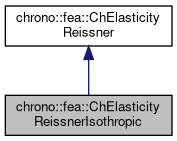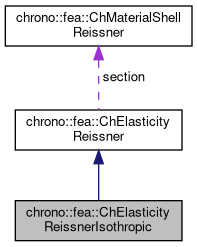chrono::fea::ChElasticityReissnerIsothropic Class Reference

## Description

Elasticity of 6-field Reissner-Mindlin shells (kinematically-exact shell theory as in Witkowski et al.) to be used in a ChMaterialShellReissner.

This class implements material properties for a layer from the Reissner theory, for the case of isotropic linear linear elastic material. This is probably the material that you need most often when using 6-field shells. Previously: ChMaterialShellReissnerIsothropic

#include <ChMaterialShellReissner.h>

Inheritance diagram for chrono::fea::ChElasticityReissnerIsothropic:[legend]
Collaboration diagram for chrono::fea::ChElasticityReissnerIsothropic:[legend]

## Public Member Functions

ChElasticityReissnerIsothropic (double E, double nu, double alpha=1.0, double beta=0.1)
Construct an isotropic material. More...

double Get_E () const
Return the elasticity moduli.

double Get_nu () const
Return the Poisson ratio.

double Get_alpha () const
Return the shear factor.

double Get_beta () const
Return the torque factor.

virtual void ComputeStress (ChVector<> &n_u, ChVector<> &n_v, ChVector<> &m_u, ChVector<> &m_v, const ChVector<> &eps_u, const ChVector<> &eps_v, const ChVector<> &kur_u, const ChVector<> &kur_v, const double z_inf, const double z_sup, const double angle)
The FE code will evaluate this function to compute per-unit-length forces/torques given the u,v strains/curvatures. More...

virtual void ComputeStiffnessMatrix (ChMatrixRef mC, const ChVector<> &eps_u, const ChVector<> &eps_v, const ChVector<> &kur_u, const ChVector<> &kur_v, const double z_inf, const double z_sup, const double angle)
Compute 12x12 stiffness matrix [Km] , that is [ds/de], the tangent of the constitutive relation per-unit-length forces/torques vs generalized strains. More...Public Attributes inherited from chrono::fea::ChElasticityReissner
ChMaterialShellReissnersection

## ◆ ChElasticityReissnerIsothropic()

 chrono::fea::ChElasticityReissnerIsothropic::ChElasticityReissnerIsothropic ( double E, double nu, double alpha = 1.0, double beta = 0.1 )

Construct an isotropic material.

Parameters
 E Young's modulus nu Poisson ratio alpha shear factor beta torque factor

## ◆ ComputeStiffnessMatrix()

 void chrono::fea::ChElasticityReissnerIsothropic::ComputeStiffnessMatrix ( ChMatrixRef mC, const ChVector<> & eps_u, const ChVector<> & eps_v, const ChVector<> & kur_u, const ChVector<> & kur_v, const double z_inf, const double z_sup, const double angle )
virtual

Compute 12x12 stiffness matrix [Km] , that is [ds/de], the tangent of the constitutive relation per-unit-length forces/torques vs generalized strains.

Parameters
 mC tangent matrix eps_u strains along u direction eps_v strains along v direction kur_u curvature along u direction kur_v curvature along v direction z_inf layer lower z value (along thickness coord) z_sup layer upper z value (along thickness coord) angle layer angle respect to x (if needed) -not used in this, isotropic

Reimplemented from chrono::fea::ChElasticityReissner.

## ◆ ComputeStress()

 void chrono::fea::ChElasticityReissnerIsothropic::ComputeStress ( ChVector<> & n_u, ChVector<> & n_v, ChVector<> & m_u, ChVector<> & m_v, const ChVector<> & eps_u, const ChVector<> & eps_v, const ChVector<> & kur_u, const ChVector<> & kur_v, const double z_inf, const double z_sup, const double angle )
virtual

The FE code will evaluate this function to compute per-unit-length forces/torques given the u,v strains/curvatures.

Parameters
 n_u forces along u direction (per unit length) n_v forces along v direction (per unit length) m_u torques along u direction (per unit length) m_v torques along v direction (per unit length) eps_u strains along u direction eps_v strains along v direction kur_u curvature along u direction kur_v curvature along v direction z_inf layer lower z value (along thickness coord) z_sup layer upper z value (along thickness coord) angle layer angle respect to x (if needed) -not used in this, isotropic

Implements chrono::fea::ChElasticityReissner.

The documentation for this class was generated from the following files:
• /builds/uwsbel/chrono/src/chrono/fea/ChMaterialShellReissner.h
• /builds/uwsbel/chrono/src/chrono/fea/ChMaterialShellReissner.cpp